# Two Years of Bayesian Bandits for E-Commerce¶## NYC College of Technology • April, 18 2019 • @AustinRochford¶#### [email protected] • [email protected]¶• Founded 2008, web optimization and personalization SaaS
• Observed 5B impressions and \$4.1B in revenue during Cyber Week 2017

#### Non-technical marketer-focused¶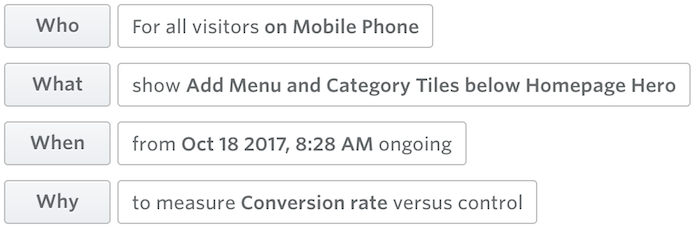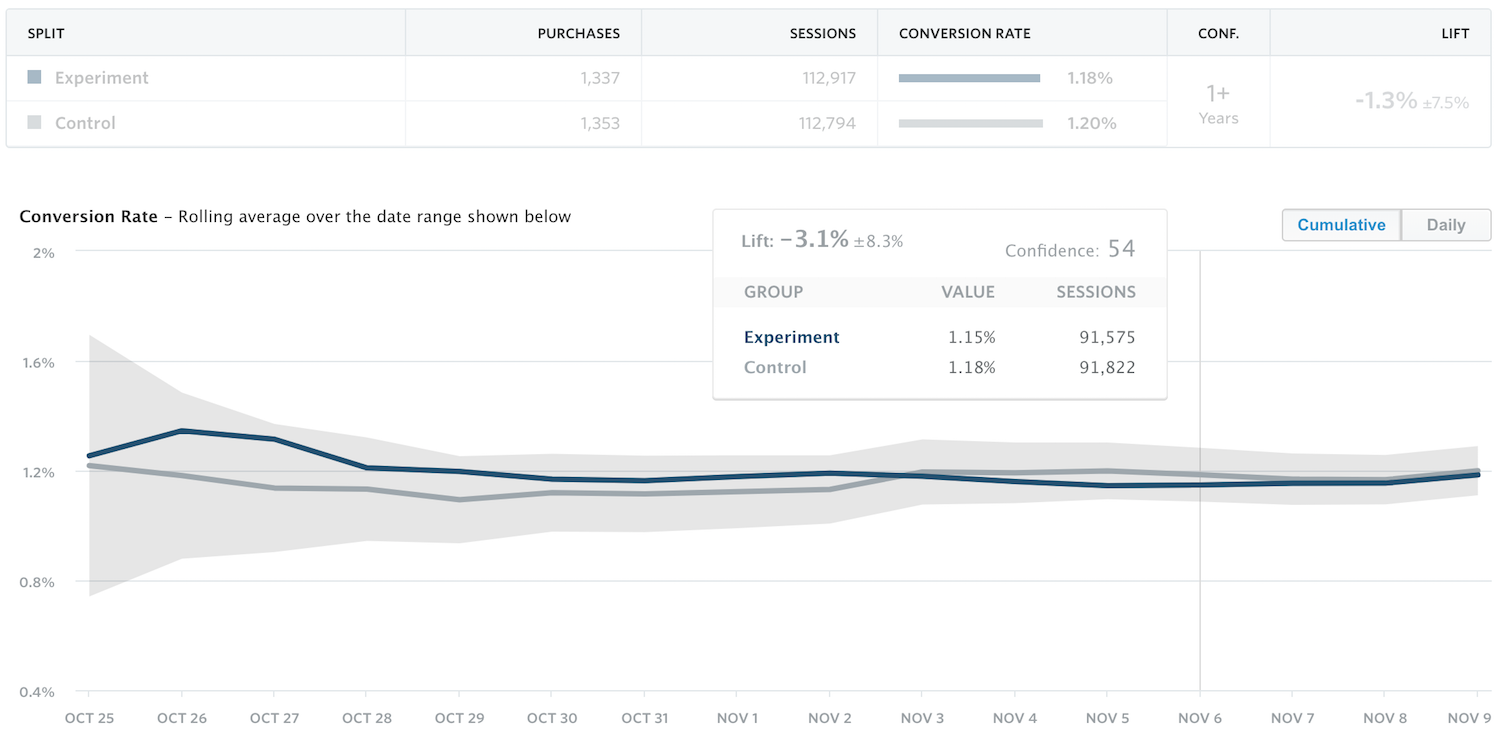### Outline¶

• Web optimization
• A/B testing
• Multi-armed bandits
• Bayesian bandits
• Thompson sampling
• Bandit bias
• Inverse propensity weighting

## Web Optimization¶

### A/B testing¶#### A/B testing machinery¶Ronald FisherWhom the Gods Would Destroy, They First Give Real-time Analytics

#### Sequential testing¶Abraham Wald

#### Sequential optimization¶### Multi-armed bandits¶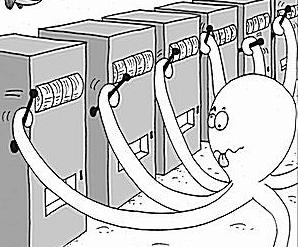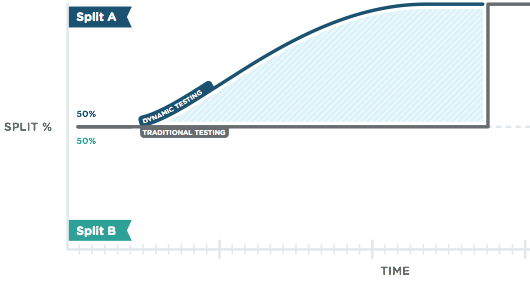#### Multi-armed bandit systems¶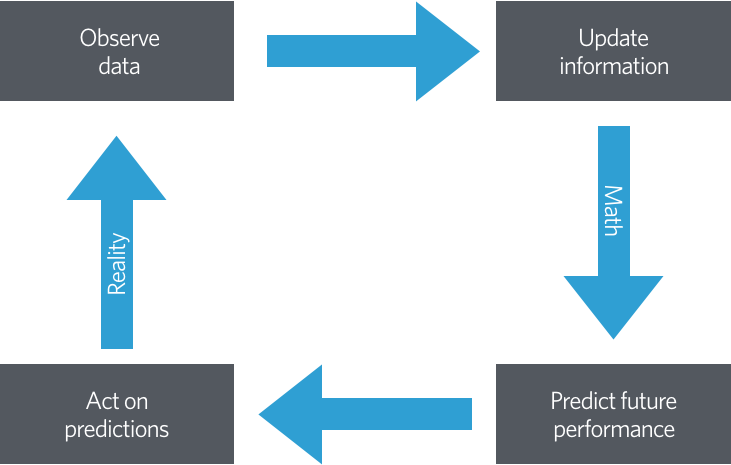## Bayesian Bandits¶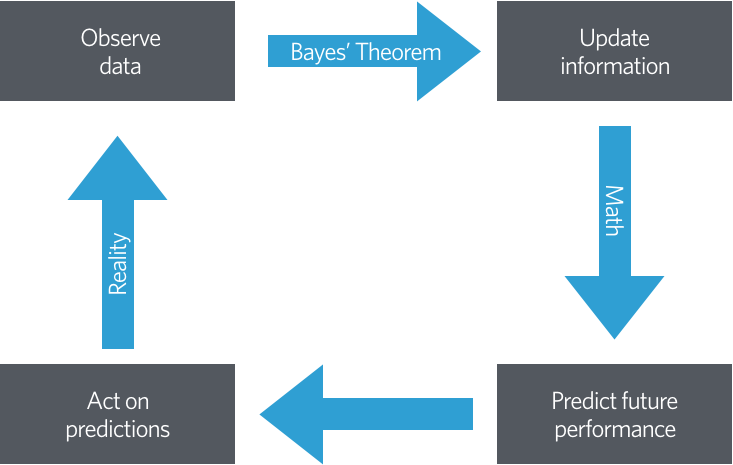### Beta-binomial model¶

\begin{align*} x_A, x_B & = \textrm{number of rewards from users shown variant } A, B \\ x_A & \sim \textrm{Binomial}(n_A, r_A) \\ x_B & \sim \textrm{Binomial}(n_B, r_B) \\ r_A, r_B & \sim \textrm{Beta}(1, 1) \end{align*}
\begin{align*} r_A\ |\ n_A, x_A & \sim \textrm{Beta}(x_A + 1, n_A - x_A + 1) \\ r_B\ |\ n_B, x_B & \sim \textrm{Beta}(x_B + 1, n_B - x_B + 1) \end{align*}

### Thompson sampling¶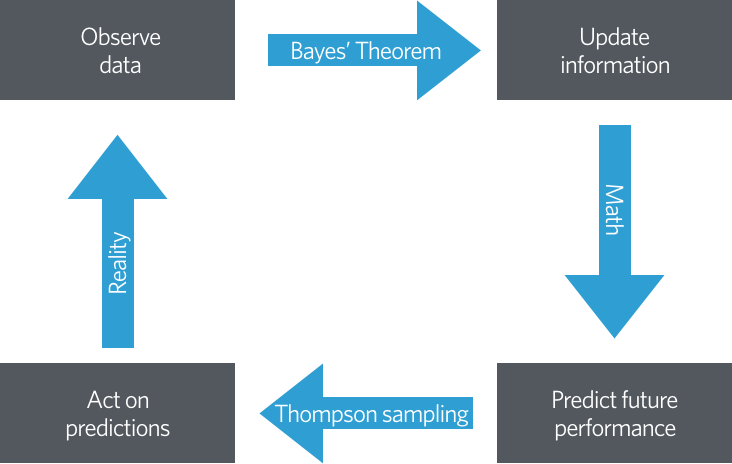Thompson sampling randomizes user/variant assignment according to the probabilty that each variant maximizes the posterior expected reward.

The probability that a user is assigned variant A is

\begin{align*} P(r_A > r_B\ |\ \mathcal{D}) & = \int_0^1 P(r_A > r\ |\ \mathcal{D})\ \pi_B(r\ |\ \mathcal{D})\ dr \\ & = \int_0^1 \left(\int_r^1 \pi_A(s\ |\ \mathcal{D})\ ds\right)\ \pi_B(r\ |\ \mathcal{D})\ dr \\ & \propto \int_0^1 \left(\int_r^1 s^{\alpha_A - 1} (1 - s)^{\beta_A - 1}\ ds\right) r^{\alpha_B - 1} (1 - r)^{\beta_B - 1}\ dr \end{align*}

#### Monte Carlo Methods¶

In :
N = 5000

x, y = np.random.uniform(0, 1, size=(2, N))

In :
fig

Out: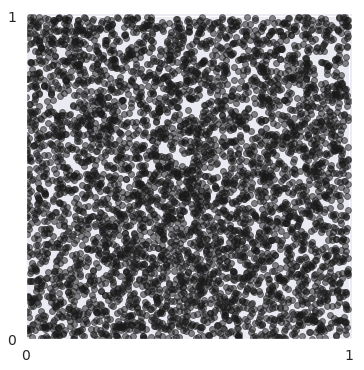In :
in_circle = x**2 + y**2 <= 1

In :
fig

Out: